# Astrophysics Collaborations Network

Weighted network of co-authorships between scientists posting preprints

Weighted network of co-authorships between scientists posting preprints on the Astrophysics E-Print Archive between Jan 1, 1995 and December 31, 1999. The edge weight indicates the strength of the collaboration between pairs of individuals.

## Examples

### Basic Examples

Retrieve the graph:

 In:=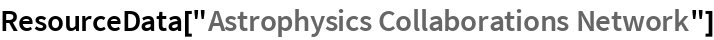Out=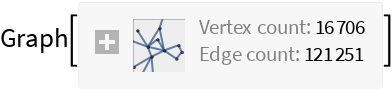View a property summary:

 In:=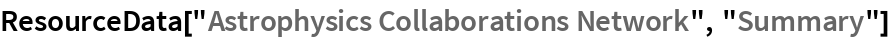Out=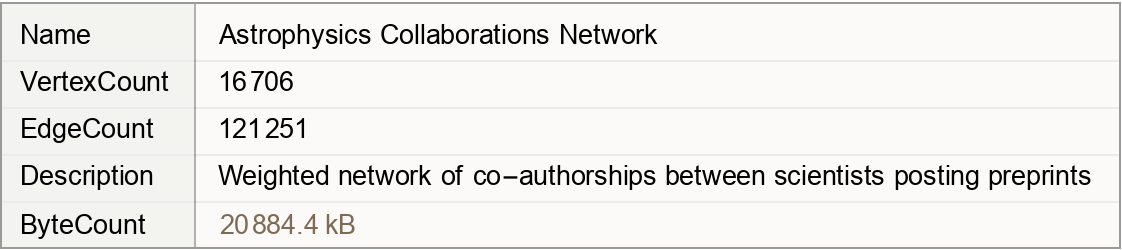### Basic Applications

Visualize the network of the neighborhood around the author with the most collaborations:

 In:=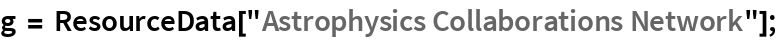In:=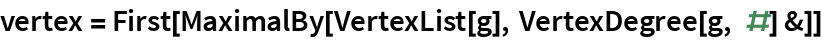Out=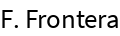In:=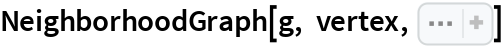Out=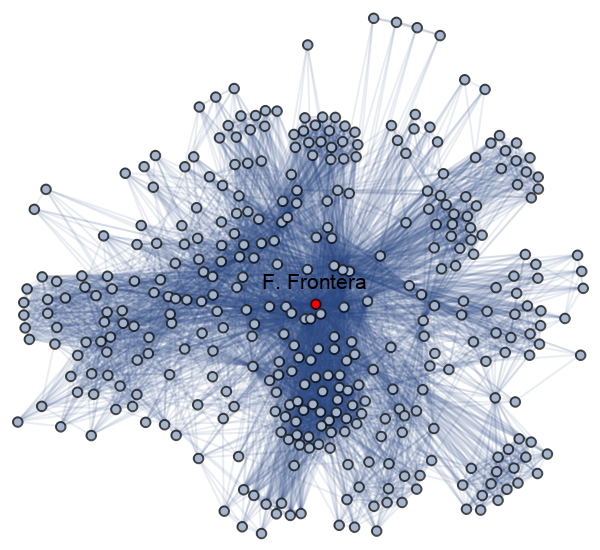Wolfram Research, "Astrophysics Collaborations Network" from the Wolfram Data Repository (2019)